# Eilenberg-MacLane space

(diff) ← Older revision | Latest revision (diff) | Newer revision → (diff)
A space, denoted by, representing the functor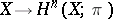, whereis a non-negative number,is a group which is commutative forand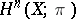is the-dimensional cohomology group of a cellular spacewith coefficients in. It exists for any suchand.
The Eilenberg–MacLane spacecan also be characterized by the condition: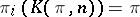forand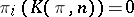for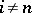, where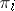is the-th homotopy group. Thus,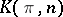is uniquely defined up to a weak homotopy equivalence. An arbitrary topological space can, up to a weak homotopy equivalence, be decomposed into a twisted product of Eilenberg–MacLane spaces (see Postnikov system). The cohomology groups of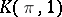coincide with those of. Eilenberg–MacLane spaces were introduced by S. Eilenberg and S. MacLane .